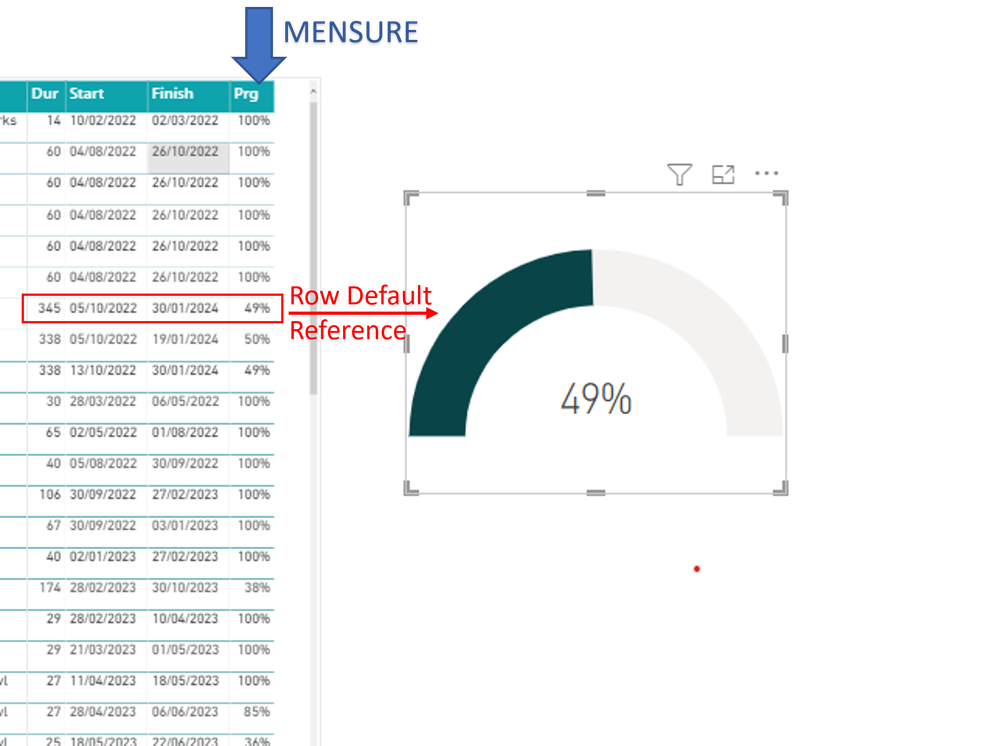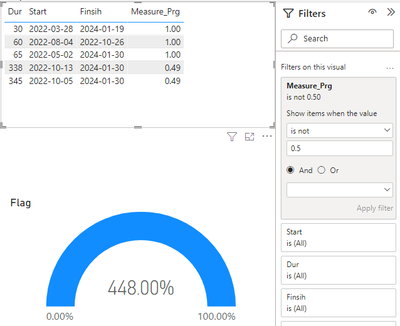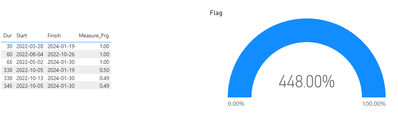cancel
Showing results for
Did you mean:Helper I

## Default Value from mensure to use Gauge

Hi Guys.
Using powerbi here I try some crazy ideas but I never find a reference, I search and search and I can't find a solution.

I want the Gauge with reference to a row, but the value comes from a measure. I couldn't use SELECTVALUE for this.

This is because in the model I have in mind, the value of the reference line is not the sum of all results. It's percentage of completion. That is, if you click on another row, the gauge will change, but without any selection, it will keep the reference row.Thanks

6 REPLIES 6Community Support

Hi  @Peleias ,

When I hide the value of 0.5 using the filter next to it, the total will still be displayed.If you want the same effect as the measure in Gauge, you can use:

``````Flag =
IF(
SELECTEDVALUE('Table'[Start]),[Measure_Prg],SUMX(FILTER(ALL('Table'),[Measure_Prg]<>0.5),[Measure_Prg]))``````Best Regards,

Liu Yang

If this post helps, then please consider Accept it as the solution to help the other members find it more quickly.Helper I

Hi @v-yangliu-msft , I'm still searching the

forum...

https://community.powerbi.com/t5/Desktop/Default-value-when-no-filter-selected/td-p/1841539

``````Comments selection =
IF (
CALCULATE (
FILTER (
)
)
)``````

Basically this is what I would like, but as I said above, my column is a measure.
Selectvalue doesn't work with measure.

TnksHelper I

Sorry @v-yangliu-msft, I think I didn't explain it right.

Percentages are based on measure with MS Project data, so max value does not apply as some activities reach 100% before the main line.

What do I need; If nothing is selected, the Gauge will show the value of the Internal Services line (in this case, 50%).In case I hide the Internal Services line later in the table, but the value will remain in the Gauge.

And when I click on the lines, they will be displayed in the Gauge.Thanks, as each answer helps me with another 10 different things in power bi.Community Support

Hi  @Peleias ,

According to the design of the power bi engine, in the table, the measure is calculated according to the context, and the value of this measure will definitely have a specified column to calculate it.

So we can select the column corresponding to this measure to perform Selectvalue.

For example, if we want to select measure=50%, we can select the corresponding column [Start]=2022-10-5I created some data:Here are the steps you can follow：

1. Create measure.

``````Measure_Prg =
MAX('Table'[Prg])``````
``````Flag =
IF(
SELECTEDVALUE('Table'[Start]),[Measure_Prg],SUMX('Table',[Measure_Prg]))``````

2. Result:

When selectedWhen not selected:Best Regards,

Liu Yang

If this post helps, then please consider Accept it as the solution to help the other members find it more quickly.Super User

@Peleias  I prefer to go with SELECTEDVALUE for this problem. Here is my solution.For the default value, I have used the MAX value.Helper I

Thanks @ghoshabhijeet

I don't think I explained it right either, sorry.

The column is a measurement. And usually the numerical value of the reference line reaches 100% last. Usually several lines reach 100% and finally the reference line reaches 100%.